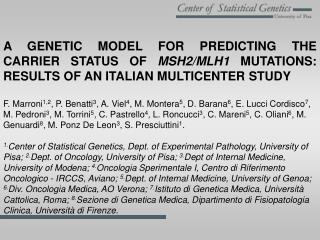DownloadDownload PresentationPredicting the carrier status of MSH2/MLH1 mutations

# Predicting the carrier status of MSH2/MLH1 mutations

Télécharger la présentation## Predicting the carrier status of MSH2/MLH1 mutations

- - - - - - - - - - - - - - - - - - - - - - - - - - - E N D - - - - - - - - - - - - - - - - - - - - - - - - - - -
##### Presentation Transcript

1. A GENETIC MODEL FOR PREDICTING THE CARRIER STATUS OF MSH2/MLH1 MUTATIONS: RESULTS OF AN ITALIAN MULTICENTER STUDY F. Marroni1,2, P. Benatti3, A. Viel4, M. Montera5, D. Barana6, E. Lucci Cordisco7, M. Pedroni3, M. Torrini5, C. Pastrello4, L. Roncucci3, C. Mareni5, C. Oliani6, M. Genuardi8, M. Ponz De Leon3, S. Presciuttini1. 1.Center of Statistical Genetics, Dept. of Experimental Pathology, University of Pisa; 2.Dept. of Oncology, University of Pisa; 3.Dept of Internal Medicine, University of Modena; 4.Oncologia Sperimentale I, Centro di Riferimento Oncologico - IRCCS, Aviano; 5.Dept. of Internal Medicine, University of Genoa; 6.Div. Oncologia Medica, AO Verona; 7.Istituto di Genetica Medica, Università Cattolica, Roma; 8.Sezione di Genetica Medica, Dipartimento di Fisiopatologia Clinica, Università di Firenze.

2. Predicting the carrier status of MSH2/MLH1 mutations Relevant Parameters • Family history • Number (proportion) of affected relatives • Age of affection • Multiple tumors • Microsatellite instability • Mutation-carriers often show MSI tumors • MSS tumors only rarely are found in mutation carriers

3. Predictive Models Empirical Model (Dutch) Empirical models rely on empirical observations in a given data-set. In this approach, families are stratified according to variables that describe their family history; regression or other approaches are used to predict the results of genetic testing. Genetic Model (AIFEG) The Mendelian models, in contrast, address the probability that a proband is a mutation carrier on the basis of explicit assumptions about the genetic parameters of the disease (allele frequencies and cancer penetrances in carriers and non-carriers), and the Mendelian rules of gene transmission.

4. Predicting the carrier status of MSH2/MLH1 mutations

5. Predicting the carrier status of MSH2/MLH1 mutations Results (Standard Versions) 68 observed mutations in 219 families Genetic Model (AIFEG) 74.3 predicted mutations (2=0.8, p=N.S) Empirical Model (Dutch) 43.8 predicted mutations (2=16.7, p < 10-4)

6. Incorporating MSI status MSS In order to keep into account the rare occurrence of MSS tumors in carriers of germ-line mutations we chose to arbitrarily set the carrier probability of subjects with MSS tumors to one hundredth of the probability evaluated without considering MS status.

7. Incorporating MSI status MSI MSI information was available in 168 families. We divided them in six groups (N=28) ordered by increasing carrier probability. For each group, we evaluated the proportion of families with MSI tumors which also carried a mutation in MSH2/MLH1, as a function of the average carrier probability. Using a least square method we found a simple analytical function to be used to correct carrier probability for the MSI information. The equation that best fitted our data was the following: PMSI=a*ln(p)+b where PMSI is the corrected probability for families in which MSI tumors are present, p is the carrier probability calculated ignoring MS status, and a and b are the parameters to be estimated. The best fit was obtained with a=0.1672 and b=0.7139 (Pearson’s correlation coefficient = 0.86).

8. Incorporating MSI status PMSI=0.1672*ln(p)+0.7139

9. Predicting the carrier status of MSH2/MLH1 mutations Results (MSI Versions) 68 observed mutations in 219 families Genetic Model (AIFEG) 73.2 predicted mutations (2=0.5, p=N.S) Empirical Model (Dutch) 50.4 predicted mutations (2=6.2, p =0.004)

10. Predicting the carrier status of MSH2/MLH1 mutations

11. Conclusions • The AIFEG model proved to be a better predictive model with respect to the Dutch model. • Both models show an improvement of performance after the information on MSI is taken into account. • After taking into account MSI information, AIFEG model still proved to be more accurate than the Dutch model • Log-likelihood of the AIFEG model was lower • The expected number of mutations was not significantly different from the observed number • AIFEG model had higher sensitivity (but lower specificity)